# Root

(diff) ← Older revision | Latest revision (diff) | Newer revision → (diff)

An-th root of a numberis a number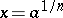whose-th power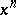is equal to.

A root of an algebraic equation over a field,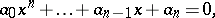is an elementbelonging toor to an extension of(cf. Extension of a field) such that whenis substituted forthe equation becomes an identity. A root of this equation is also called a root or zero of the polynomial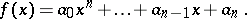Ifis a root of a polynomial, thenis divisible (without remainder) by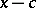(see Bezout theorem). Every polynomialwith real or complex coefficients has at least one root (hence as many roots as its degree, counting multiplicities). The polynomialmay be expressed as a productwhere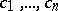are its roots. If some of the rootsofare equal, their common value is called a multiple root (if a root occurstimes,is called the multiplicity of that root).

A root of unity is an element of a fieldsatisfying the equation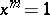for some natural number. The roots of unity form a subgroup of the multiplicative group of. Conversely, all elements of any finite subgroup of the multiplicative group of a fieldare roots of unity (cf. Fermat little theorem) and the subgroup itself is cyclic. This is true, in particular, for the subgroup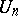of all roots of unity of a given degreecontained in the algebraic closure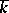of, i.e. the subgroup of all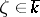satisfying the equation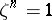. Ifis relatively prime to the characteristic of(or if the characteristic is 0), then the groupis of orderand its generators are known as primitive-th roots of unity. The number of such roots inis given by the Euler function, i.e. the number of residues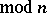which are relatively prime to. In a field of characteristicthere are no-th roots of unity other than 1.

If the fieldis finitely generated over its prime subfield, then the number of roots of unity inis finite.

In the field of complex numbers, a numberis an-th root of unity if and only ifand, whereandare integers, i.e. if and only ifin this case the primitive roots of unity are exactly those for which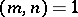. In the complex plane, the-th roots of unity coincide with the vertices of the regular-gon inscribed in the unit circle; this explains the connection of roots of unity with the problem of squaring the circle (construction of polygons, cf. Geometric constructions).

Roots of unity appear in number theory as the values of various important number-theoretical functions (Abelian numerical characters; Legendre symbol; Möbius function; norm-residue symbol; etc.). In field theory and algebraic number theory an important position is occupied by fields obtained by adjunction of roots of unity to some ground field (see Cyclotomic field; Cyclotomic extension; Kummer extension).

How to Cite This Entry:
Root. Encyclopedia of Mathematics. URL: http://encyclopediaofmath.org/index.php?title=Root&oldid=17528
This article was adapted from an original article by L.V. Kuz'min (originator), which appeared in Encyclopedia of Mathematics - ISBN 1402006098. See original article